# 强化学习之MDP马尔科夫决策过程

What is the relationship between Markov Decision Processes and Reinforcement Learning?

In Reinforcement Learning (RL), the problem to resolve is described as a Markov Decision Process (MDP). Theoretical results in RL rely on the MDP description being a correct match to the problem. If your problem is well described as a MDP, then RL may be a good framework to use to find solutions. That does not mean you need to fully describe the MDP (all the transition probabilities), just that you expect an MDP model could be made or discovered.

Conversely, if you cannot map your problem onto a MDP, then the theory behind RL makes no guarantees of any useful result.

One key factor that affects how well RL will work is that the states should have the Markov property - that the value of the current state is enough knowledge to fix immediate transition probabilities and immediate rewards following an action choice. Again you don’t need to know in advance what those are, just that this relationship is expected to be reliable and stable. If it is not reliable, you may have a POMDP. If it is not stable, you may have a non-stationary problem. In either case, if the difference from a more strictly defined MDP is small enough, you may still get away with using RL techniques or need to adapt them slightly.

The general relationship between RL and MDP is that RL is a framework for solving problems that can be expressed as MDPs.

MDP是当前强化学习理论推导的基石，对强化学习来说，一般以马尔科夫决策过程作为形式化问题的手段。也就是说，对于目前的绝大部分强化学习算法，只有可以将问题抽象为MDP的才可以确保算法的性能（收敛性，效果等），对于违背MDP的问题并不一定确保算法有效，因为其数学公式都是基于MDP来进行推导的。

## 马尔科夫性## 马尔科夫过程

1. S是有限状态集合
2. P是状态转移概率矩阵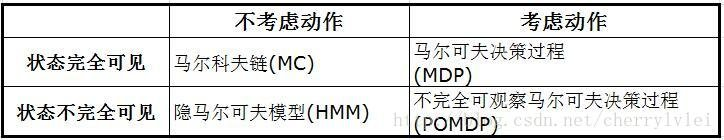### 状态转移矩阵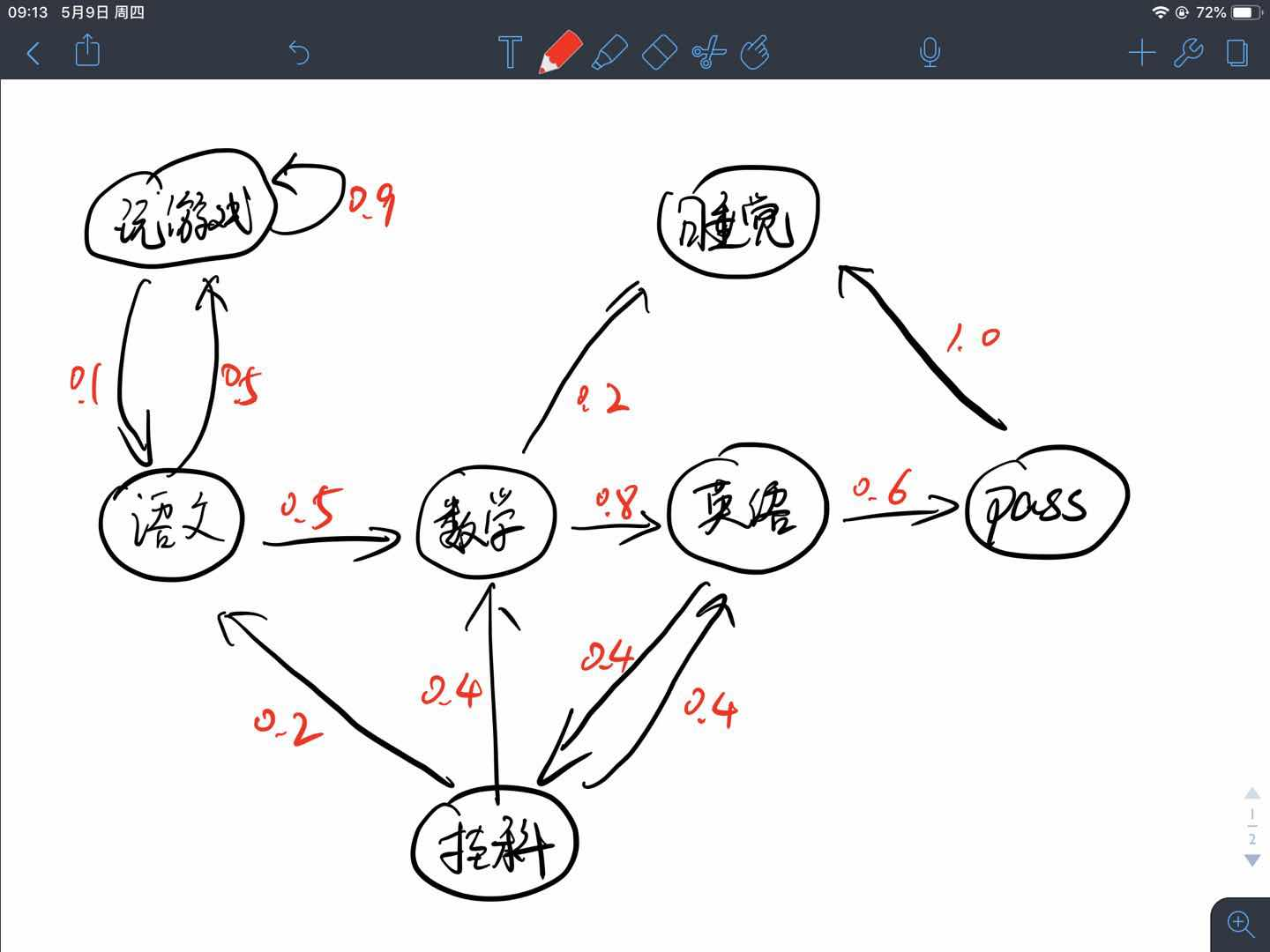### 马尔科夫链与Episode

Episode可以翻译为片段、情节、回合等，在强化学习问题中，一个Episode就是一个马尔科夫链，根据状态转移矩阵可以得到许多不同的episode，也就是多个马尔科夫链。

1. 如果一个任务总能达到终态，结束任务或者开启下一轮任务，那么这个任务就被称为回合任务，也就是episode任务。例如，让一个智能体学习如何下围棋，围棋棋盘只有那么大，游戏定会终局，所以是一个回合式任务。
2. 如果一个任务可以无限持续下去，永远不会结束，即永远在训练当中，那么这个任务就被称为连续性任务。例如，教会一辆车能够进行自动驾驶就是一个连续性任务，不要钻牛角尖说能源会耗尽，车子会磨损，我们只聚焦问题与环境本身，不涉及其他非稳定因素。

1. $B-C-D-E-C-G$，即“学语文→数学→英语→考试没通过,挂科→继续学数学→睡觉”
2. $B-A-A-…-A-B-C-G$，即“学语文→玩王者荣耀→玩刺激战场→玩OverCooker→玩守望先锋→玩英雄联盟→玩CS:GO→…→看一会儿数学→睡觉”。（仿佛就是我自己嘛！）

## 马尔科夫奖励过程

1. $S$代表了状态的集合(也是维度有限的)
2. $P$描述了状态转移矩阵$\mathcal{P}_{ss’}=\mathbb{P}[S_{t+1}=s’|S_{t}=s]$
3. $R$表示奖励函数，$R(s)$描述了在状态$s$下的期望(立即)奖励，$\mathcal{R}(s)=\mathbb{E}[R_{t+1}|S_{t}=s]$
4. $\gamma$表示衰减因子,即discounted factor,$\gamma\in[0,1]$

$\gamma$是用来计算累计奖励回报的,表示我们有多看中现在或者未来,为什么这么说呢?假设我们现在要计算一个episode始态$S_{0}$的奖励值$V(S_{0})$,不涉及具体公式推导的说,我们应该把$S_{0}$状态后续的奖励全部加和,这样就得到了对始态$S_{0}$的值估计,这些后续奖励的值的权重都是1,或者说此时$\gamma=1$,但是当前状态对很多步之后的状态未必影响很大,我们这样计算过来并不能完全表示一个状态的值,那么我们应当顺势减少距离远的状态的权重,此时$\gamma\lt1$

• 当$\gamma=0$时,状态$S$的值完全由其转移的期望立即奖励表示,即一点都不关心未来
• 当$\gamma=1$时,状态$S$的值由以当前状态为始态,运行至终态所得到的所有立即奖励加和的值表示,即未来与现在同等重要
• 当$0 \lt\gamma \lt1$时,状态$S$的值是前两个模式的trade-off,即对未来看重的程度由$\gamma$决定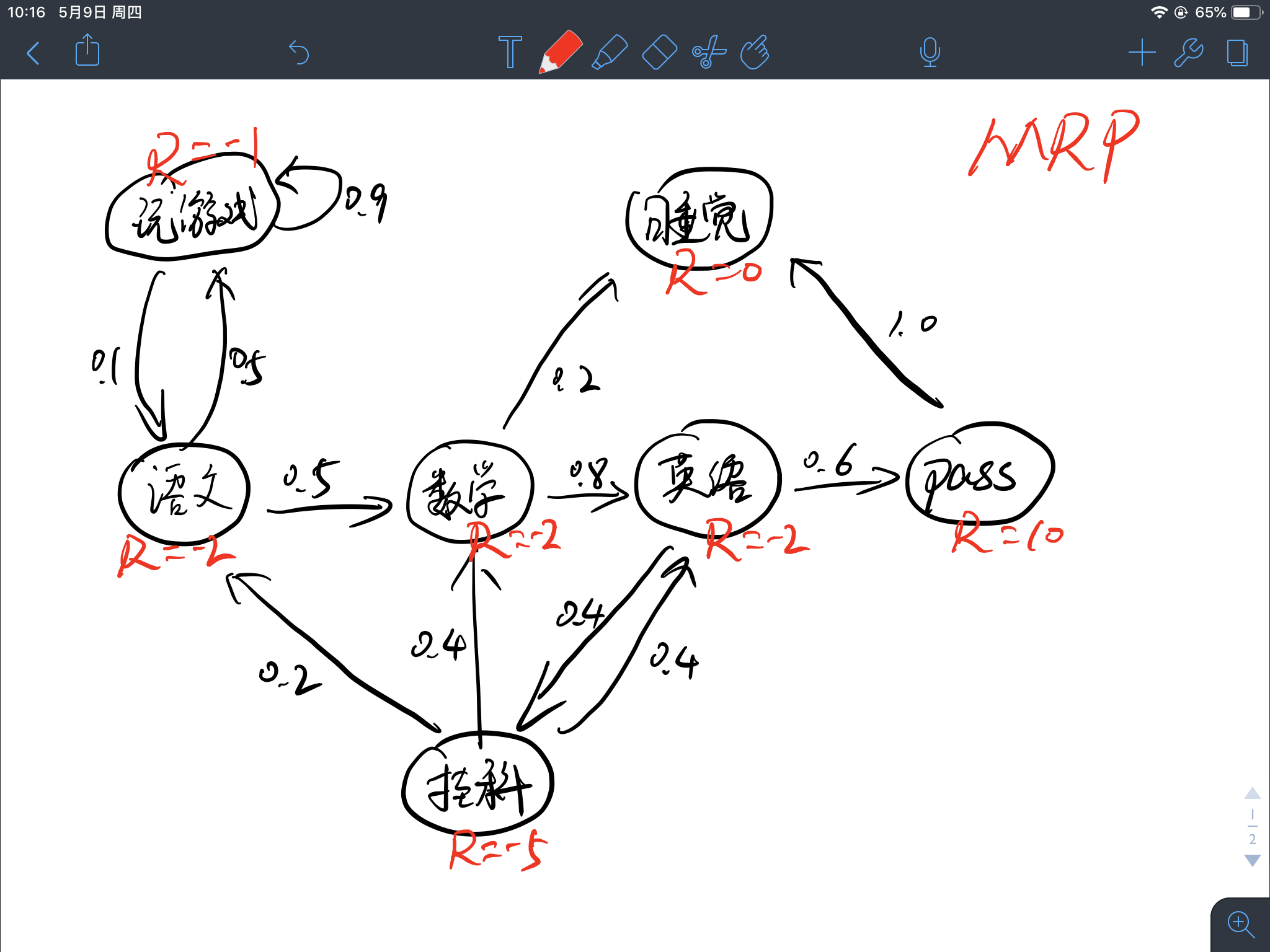## 马尔科夫决策过程

1. $S$代表了状态的集合(也是维度有限的)
2. $A$代表了决策过程中动作的集合(维度有限的)
3. $P$描述了状态转移矩阵$\mathcal{P}_{ss’}^{a}=\mathbb{P}[S_{t+1}=s’|S_{t}=s,A_{t}=a]$
4. $R$表示奖励函数，$R(s)$描述了在状态$s$下执行某动作的期望(立即)奖励，$\mathcal{R}(s,a)=\mathbb{E}[R_{t+1}|S_{t}=s,A_{t}=a]$
5. $\gamma$表示衰减因子,即discounted factor,$\gamma\in[0,1]$

MDPs是一个从交互中达成目标的强化学习问题的一个直接的框架。学习者和决策者叫做Agent。Agent进行交互的其它一切Agent之外的东西都叫做环境。Agent不断的选择动作，而环境也给出相应的反应，并且向Agent表现出新的状态。环境同时也给出一个数值作为反馈。Agent的目标就是通过选择不同的Action来最大化这个反馈值。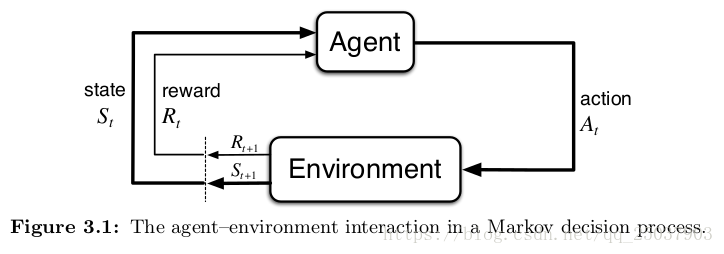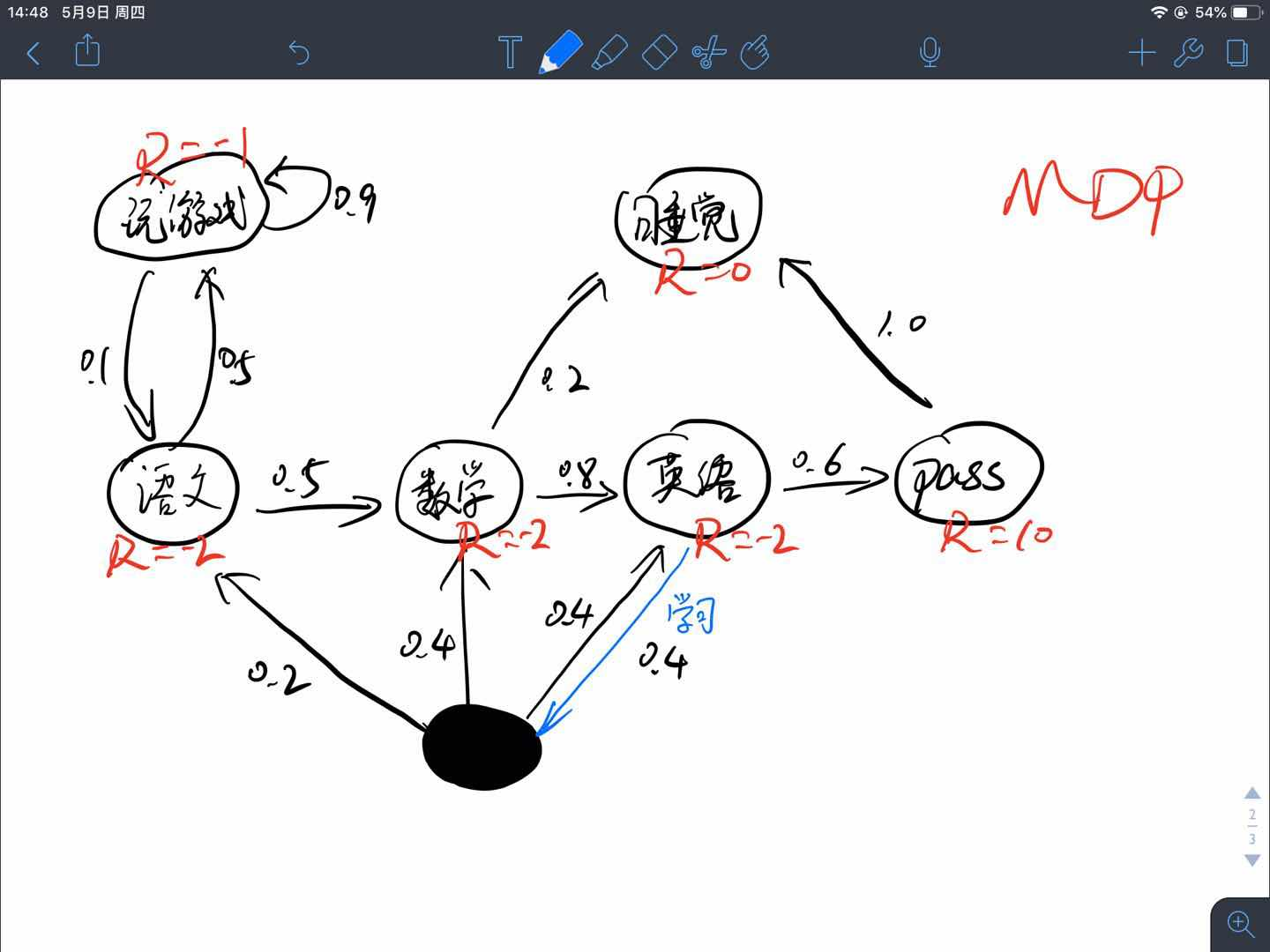-------------本文结束感谢您的阅读-------------

0%